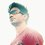# Any shortcut?

Coefficient of $x^{49}$ in expansion of $(x+1)(x+2)(x+3)\dots(x+100)$

or you can generalise it for any power coefficient?Note by Aman Rajput
5 years, 10 months ago

This discussion board is a place to discuss our Daily Challenges and the math and science related to those challenges. Explanations are more than just a solution — they should explain the steps and thinking strategies that you used to obtain the solution. Comments should further the discussion of math and science.

When posting on Brilliant:

• Use the emojis to react to an explanation, whether you're congratulating a job well done , or just really confused .
• Ask specific questions about the challenge or the steps in somebody's explanation. Well-posed questions can add a lot to the discussion, but posting "I don't understand!" doesn't help anyone.
• Try to contribute something new to the discussion, whether it is an extension, generalization or other idea related to the challenge.

MarkdownAppears as
*italics* or _italics_ italics
**bold** or __bold__ bold
- bulleted- list
• bulleted
• list
1. numbered2. list
1. numbered
2. list
Note: you must add a full line of space before and after lists for them to show up correctly
paragraph 1paragraph 2

paragraph 1

paragraph 2

[example link](https://brilliant.org)example link
> This is a quote
This is a quote
    # I indented these lines
# 4 spaces, and now they show
# up as a code block.

print "hello world"
# I indented these lines
# 4 spaces, and now they show
# up as a code block.

print "hello world"
MathAppears as
Remember to wrap math in $$ ... $$ or $ ... $ to ensure proper formatting.
2 \times 3 $2 \times 3$
2^{34} $2^{34}$
a_{i-1} $a_{i-1}$
\frac{2}{3} $\frac{2}{3}$
\sqrt{2} $\sqrt{2}$
\sum_{i=1}^3 $\sum_{i=1}^3$
\sin \theta $\sin \theta$
\boxed{123} $\boxed{123}$

Sort by:

me also waiting for this

- 5 years, 9 months ago

then please share it again and again

- 5 years, 9 months ago

- 5 years, 10 months ago

Generalize? Yes, but the formula is too long and worth writing it down.

To solve this question, look at a similar question here.

- 5 years ago

- 5 years ago

I'm pessimistic that the formula will be helpful. It's like trying to remember all 4 quartic formulas.

- 5 years ago

okay you can write the formula here

- 5 years ago

Look at the solutions in the link that I've given. That's the general approach.

- 5 years ago

Very simple . Use vietta's to generalise . Like : let f(x)= (x+1)(x+2)(x+3)......(x+n) then , f(x)= x^n + (1+2+3+...n).x^(n-1) + (1.2+1.3+1.4+...1.n+ 2.3+2.4+...2.n+3.4+3.5 +...).x^(n-2) +... I hope it is clear now!

- 4 years, 10 months ago

so tell me the answer of the my question... i know that what you have written .

- 4 years, 10 months ago